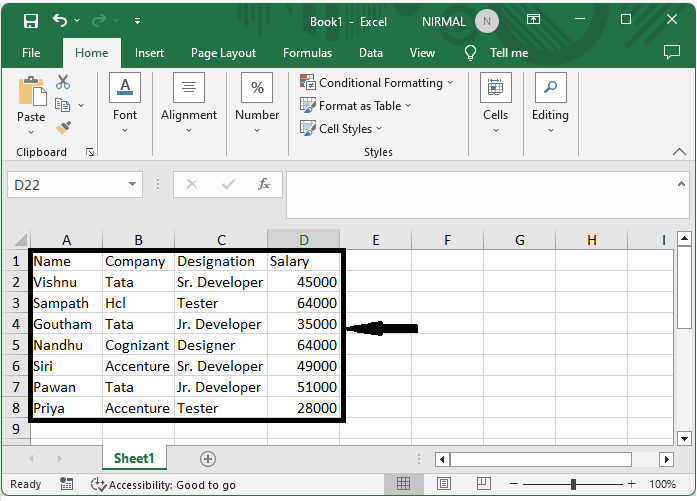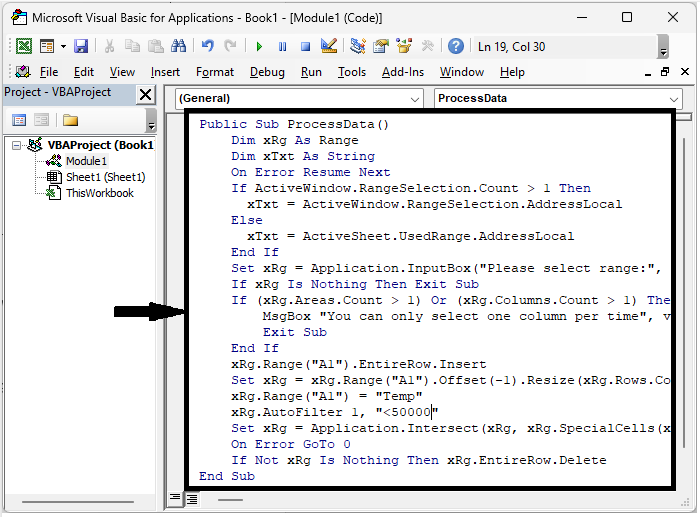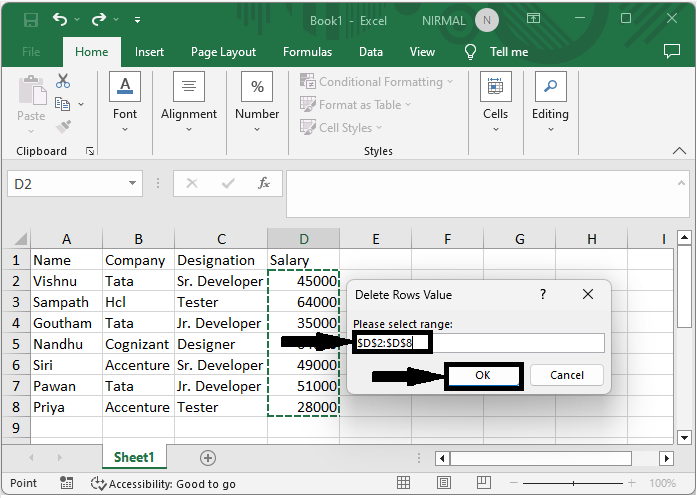# How to Delete Rows if Cell is Greater or Less Than a Certain Value in Excel

Excel is a great tool for organising and analysing data, and it is occasionally necessary to delete rows that do not satisfy particular criteria. When working with huge datasets or filtering out data that does not reach certain thresholds, deleting rows based on a condition can be quite useful. This article will concentrate on deleting rows when a cell within a specific column meets a greater or lesser than criteria.

## Delete Rows if a Cell is Greater/Less Than a Certain Value

Here we will use the VBA application to complete the task. So let us see a simple process to know how you can delete rows if a cell is greater or less than a certain value in Excel.

### Step 1

Consider an Excel sheet where the data in the sheet is similar to the below image.First, use Alt + F11 to open the VBA application.

### Step 2

Then click on Insert and select Module, then copy the below code into the text box.

### Example

Public Sub ProcessData()
Dim xRg As Range
Dim xTxt As String
On Error Resume Next
If ActiveWindow.RangeSelection.Count > 1 Then
Else
End If
Set xRg = Application.InputBox("Please select range:", "Delete Rows Value",
xTxt, , , , , 8)
If xRg Is Nothing Then Exit Sub
If (xRg.Areas.Count > 1) Or (xRg.Columns.Count > 1) Then
MsgBox "You can only select one column per time", vbInformation, "Delete
Rows Value"
Exit Sub
End If
xRg.Range("A1").EntireRow.Insert
Set xRg = xRg.Range("A1").Offset(-1).Resize(xRg.Rows.Count + 1)
xRg.Range("A1") = "Temp"
xRg.AutoFilter 1, "<50000"
Set xRg = Application.Intersect(xRg, xRg.SpecialCells(xlCellTypeVisible))
On Error GoTo 0
If Not xRg Is Nothing Then xRg.EntireRow.Delete
End SubIn the code, <200 represents that if the value is less than 50000, then rows will be deleted.

### Step 3

Then click F5 to run the module, select the range of cells where you have a certain value, and click OK to complete the task.

F5 > Select cells > Ok.## Conclusion

In this tutorial, we have used a simple example to demonstrate how you can delete rows if a cell is greater or less than a certain value in Excel to highlight a particular set of data.

Updated on: 12-Jul-2023

112 Views